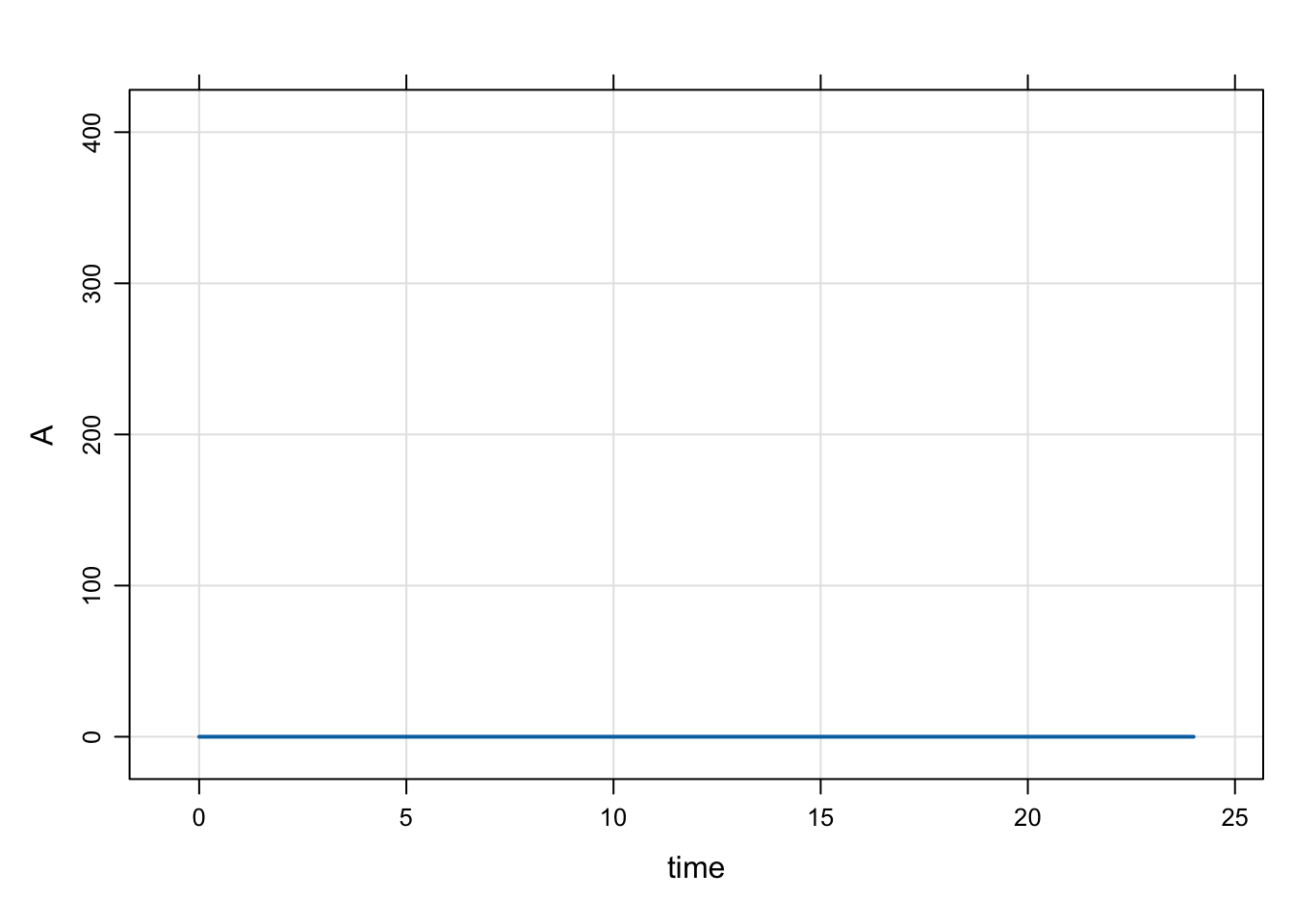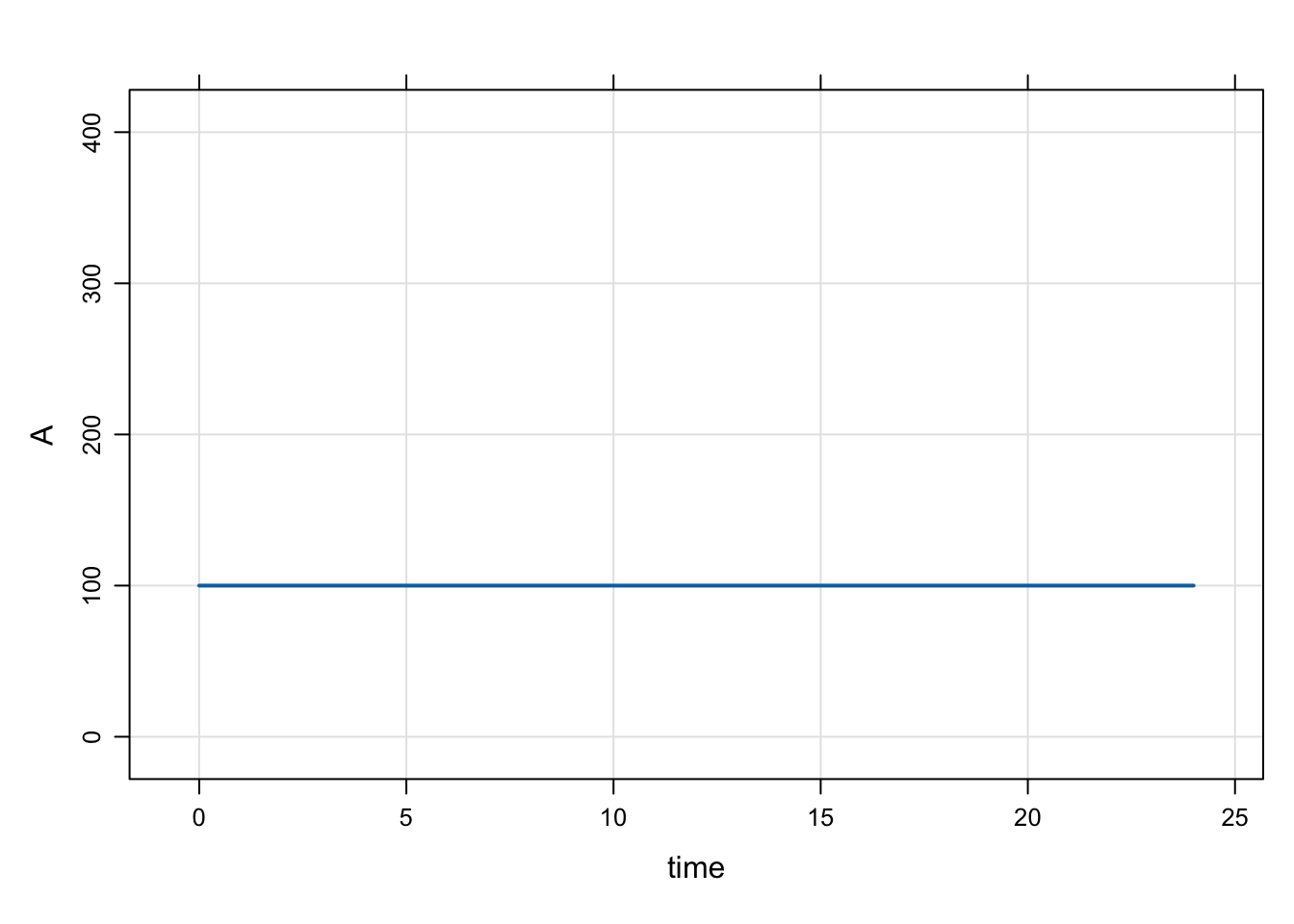There are two commonly-used ways to set initial conditions: in $MAIN and in the initial condition list. Set initials in$MAIN

For a compartment called CMT, there is a variable available to you called CMT_0 that you can use to set the initial condition of that compartment in $MAIN. For example: code <- '$PARAM KIN = 200, KOUT = 50

$CMT RESP$MAIN
RESP_0 = KIN/KOUT;
'

'

This method gets us the same result as the previous example, however the initial condition now is not a derived value, but it is coded as a number. Alternatively, you could declare a compartment via $CMT and update later (see next). We can update this value later like this mod <- mcode("init_up", code) init(mod) . . Model initial conditions (N=1): . name value . name value . RESP (1) 4 | . ... . init(mod, RESP=8) %>% init . . Model initial conditions (N=1): . name value . name value . RESP (1) 8 | . ... . This method is commonly used to set initial conditions in large QSP models where the compartment starts out as some known or assumed steady state value. Don’t use initial conditions as a dosing mechanism Using an initial condition to put a starting dose in a compartment is not recommended. Always use a dosing event for that. Long answer The following is from a wiki post I did on the topic. It’s pedantic. But hopefully helpful to learn what mrgsolve is doing for those who want to know. • mrgsolve keeps a base list of compartments and initial conditions that you can update either from R or from inside the model specification • When you use$CMT, the value in that base list is assumed to be 0 for every compartment
• mrgsolve will by default use the values in that base list when starting the problem
• When only the base list is available, every individual will get the same initial condition
• You can override this base list by including code in $MAIN to set the initial condition • Most often, you do this so that the initial is calculated as a function of a parameter • For example,$MAIN RESP_0 = KIN/KOUT; when KIN and KOUT have some value in $PARAM • This code in$MAIN overwrites the value in the base list for the current ID
• For typical PK/PD type models, we most frequently initialize in $MAIN • This is equivalent to what you might do in your NONMEM model • For larger systems models, we often just set the initial value via the base list Make a model only to examine init behavior Note: IFLAG is my invention only for this demo. The demo is always responsible for setting and interpreting the value (it is not reserved in any way and mrgsolve does not control the value). For this demo • Compartment A initial condition defaults to 0 • Compartment A initial condition will get set to BASE only if IFLAG > 0 • Compartment A always stays at the initial condition (the system doesn’t advance) code <- '$PARAM BASE=200, IFLAG = 0

$CMT A$MAIN
if(IFLAG > 0) A_0 = BASE;

$ODE dxdt_A = 0; ' mod <- mcode("init_long",code) dplot <- function(x) plot(x,ylim=c(0,400)) Check the initial condition init(mod) . . Model initial conditions (N=1): . name value . name value . A (1) 0 | . ... . Note: • We used$CMT in the model spec; that implies that the base initial condition for A is set to 0
• In this chunk, the code in $MAIN doesn’t get run because IFLAG is 0 • So, if we don’t update something in$MAIN the initial condition is as we set it in the base list
mod %>% mrgsim %>% dplotNext, we update the base initial condition for A to 100

Note:

• The code in $MAIN still doesn’t get run because IFLAG is 0 mod %>% init(A = 100) %>% mrgsim %>% dplotNow, turn on IFLAG Note: • Now, that code in$MAIN gets run
• A_0 is set to the value of BASE
mod %>% param(IFLAG=1) %>% mrgsim %>% dplotmod %>% param(IFLAG=1, BASE=300) %>% mrgsim %>% dplotExample PK/PD model with initial condition

Just to be clear, there is no need to set any sort of flag to set the initial condition.

code <- '
$PARAM AUC=0, AUC50 = 75, KIN=200, KOUT=5$CMT RESP

$MAIN RESP_0 = KIN/KOUT;$ODE
dxdt_RESP = KIN*(1-AUC/(AUC50+AUC)) - KOUT*RESP;
'
mod <- mcode("init_long2", code)

The initial condition is set to 40 per the values of KIN and KOUT

mod %>% mrgsim %>% plotEven when we change RESP_0 in R, the calculation in $MAIN gets the final say mod %>% init(RESP=1E9) %>% mrgsim . Model: init_long2 . Dim: 25 x 3 . Time: 0 to 24 . ID: 1 . ID time RESP . 1: 1 0 40 . 2: 1 1 40 . 3: 1 2 40 . 4: 1 3 40 . 5: 1 4 40 . 6: 1 5 40 . 7: 1 6 40 . 8: 1 7 40 Calling init will let you check to see what is going on • It’s a good idea to get in the habit of doing this when things aren’t clear • init first takes the base initial condition list, then calls$MAIN and does any calculation you have in there; so the result is the calculated initials
init(mod)
.
.  Model initial conditions (N=1):
.  name       value . name    value
.  RESP (1)   40    | . ...   .
mod %>% param(KIN=100) %>% init
.
.  Model initial conditions (N=1):
.  name       value . name    value
.  RESP (1)   20    | . ...   .

Set initial conditions via idata

Go back to house model

mod <- mrgsolve:::house()
init(mod)
.
.  Model initial conditions (N=3):
.  name       value . name       value
.  CENT (2)   0     | RESP (3)   50
.  GUT (1)    0     | . ...      .

Notes

• In idata (only), include a column with CMT_0 (like you’d do in $MAIN). • When each ID is simulated, the idata value will override the base initial list for that subject. • But note that if CMT_0 is set in$MAIN, that will override the idata update.
idata <- expand.idata(CENT_0 = seq(0,25,1))

.   ID CENT_0
. 1  1      0
. 2  2      1
. 3  3      2
. 4  4      3
. 5  5      4
. 6  6      5
out <-
mod %>%
idata_set(idata) %>%
mrgsim(end=40)
plot(out, CENT~.)mrgsolve: mrgsolve.github.io | metrum research group: metrumrg.com